# Static maps with matplotlib¶

Before we start you need to download (and then extract) the dataset zip-package used during this lesson from this link.

You should have following Shapefiles in the `Data` folder:

• population_square_km.shp

• schools_tartu.shp

```population_square_km.shp.xml  schools_tartu.cpg
population_square_km.shx      schools_tartu.csv
population_square_km.sbn
population_square_km.sbx
population_square_km.shp
```

## Static maps in Geopandas¶

We have already seen during the previous lessons quite many examples how to create static maps using Geopandas.

Thus, we won’t spend too much time repeating making such maps but let’s create one with more layers on it.

Let’s create a static neighbourhood map with roads and schools line on it and population density as a background.

First, we need to read the data.

```import geopandas as gpd
import matplotlib.pyplot as plt
%matplotlib inline

# Filepaths
grid_fp = "population_square_km.shp"

schools_fp = "schools_tartu.shp"

```

Then, we need to be sure that the files are in the same coordinate system. Let’s use the crs of our travel time grid.

```gridCRS = grid.crs
schools['geometry'] = schools['geometry'].to_crs(crs=gridCRS)
```
```# in Jupyter Notebook don't forget to enable the inline plotting magic
import matplotlib.pyplot as plt
%matplotlib inline
```

Let’s check the histgram first:

```# Plot
grid.hist(column="Population", bins=100)
plt.title("Amount of population km2 Tartumaa histogram")
plt.tight_layout()
```Finally we can make a visualization using the `.plot()` -function in Geopandas. The `.plot()` function takes all the matplotlib parameters where appropriate. For example we can adjust various parameters

• `ax` if used, then can indicate a joint plot axes onto which to plot, used to plot several times (several layers etc) into the same plot (using the same axes, i.e. x and y coords)

• `column` which dataframe column to plot

• `linewidth` if feature with an outline, or being a line feature then line width

• `markersize` size of point/marker element to plot

• `color` colour for the layers/feature to plot

• `cmap` colormaps (*cmap* - parameter)

• `alpha` transparency 0-1

• `legend` True/False show the legend

• `scheme` one of 3 basic classification schemes (“quantiles”, “equal_interval”, “fisher_jenks”), beyond that use PySAL explicitly

• `k` number of classes for above scheme if used.

• `vmin` indicate a minimal value from the data column to be considered when plotting (also affects the classification scheme), can be used to “normalise” several plots where the data values don’t aligh exactly

• `vmax` indicate a maximal value from the data column to be considered when plotting (also affects the classification scheme), can be used to “normalise” several plots where the data values don’t aligh exactly

```fig, ax = plt.subplots(figsize=(15, 13))

# Visualize the population density into 5 classes using "Quantiles" classification scheme
# Add also a little bit of transparency with `alpha` parameter
# (ranges from 0 to 1 where 0 is fully transparent and 1 has no transparency)
grid.plot(column="Population", ax=ax, linewidth=0.03, cmap="Reds", scheme="quantiles", k=5, alpha=0.8, legend=True)

# (use ax parameter to define the map on top of which the second items are plotted)

# Add schools on top of the previous map
schools.plot(ax=ax, color="cyan", markersize=9.0)

# Remove the empty white-space around the axes
plt.title("population km2 Tartumaa in relation to schools and major roads")

ax.set_ylabel('Northing in EPSG:3301 Estonian grid (metres)')
ax.set_xlabel('Easting in EPSG:3301 Estonian grid (metres)')

plt.tight_layout()
``````outfp = "static_map.png"
plt.savefig(outfp, dpi=300)
```

This kind of approach can be used really effectively to produce large quantities of nice looking maps (though this example of ours isn’t that pretty yet, but it could be) which is one of the most useful aspects of coding and what makes it so important to learn how to code.

## EarthPy DEM and Hillshade raster with normal GeoDataFrame vector data¶

We introduced EarthPy in the lesson about working with raster data. EarthPy also explicitly relies on matplotlib for plotting operations. However, most of EarthPy’s practical plotting operations aim at raster data. This adds some neat features on top of rasterio.plot for convenience again.

In this example I demonstrate how to combine raster plots from EarthPy and our standard GeoPandas vector plots:

```import numpy as np
import matplotlib.pyplot as plt
import earthpy as et
import earthpy.spatial as es
import earthpy.plot as ep
import rasterio as rio

dtm = '../L5/dem.tif'

# Open the DEM with Rasterio
src = rio.open(dtm)
```

Now we load the vector datasets. polygons and points. We will also visualize the point-in-polygon query for the Idaoja subcatchment.

```import geopandas as gpd

subcatch = catchments.loc[catchments['NAME_1']=='Idaoja']

# protected species under class 3 monitoring sightings
species_fp = "../L3/category_3_species_porijogi.gpkg"

```

The actual spatial analysis is now not so scary anymore.

```pip_mask = species_data.within(subcatch.loc[0, 'geometry'])
```

However, for legends we will plot the elevation colorbar from EarthPy plot, but we should also add information about the observed species records. So we will stitch together a custom legend info:

```import matplotlib.patches as mpatches
import matplotlib.pyplot as plt
import collections

patchList = []

data_key = mpatches.Patch(color="gold", label="Selected Idaoja species")

patchList.append(data_key)
```

For effects, we add the hillshade to the plot. It gives us some nice tactile pattern for the DEM image.

```hillshade = es.hillshade(elevation)
```

The tricky bit to combine the raster and vector plots here in this example is that they have different spatial extents. They should be in the same CRS anyway, but in order to align the gridded, array-based images with the discrete vector shapes is in providing spatial context.

For this we need to provide a tuple/list, that holds minx, maxx, miny and maxy coordinate values like so:

```# to define the bounds of the map (important for the raster layers plot)
extent = (src.bounds.left, src.bounds.right, src.bounds.bottom, src.bounds.top)
```

Now we can combine these plots nicely together.

```fig, ax = plt.subplots(figsize=(12,8))

ep.plot_bands(elevation, ax=ax, cmap="terrain", extent=extent, title="Lidar Digital Elevation Model (DEM)\n overlayed on top of a hillshade" )

ep.plot_bands(hillshade, cmap='Greys', extent=extent, ax=ax, alpha=0.3, cbar=None)

catchments.plot(ax=ax, facecolor="none", edgecolor='black', lw=0.7)

subcatch.plot(ax=ax, facecolor='red', alpha=0.2)

pip_data.plot(ax=ax, color='gold', markersize=10, legend=True)

plt.legend(handles=patchList, loc='lower center', bbox_to_anchor=(-0.5, -0.1), ncol=1)

plt.tight_layout()

```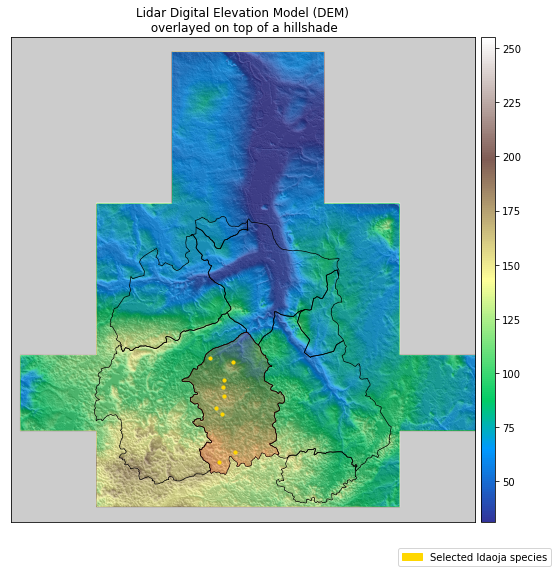## Special plots types with Geoplot¶

https://residentmario.github.io/geoplot/gallery/index.html

Note

From the website:

Geoplot is a high-level Python geospatial plotting library designed for data scientists and geospatial analysts that just want to get things done. It is an extension to cartopy and matplotlib which makes mapping easy.

Geoplot provides a few handy plot types, that would be very complicated to manually describe in matplotlib. In the following some examples are shown, that use data from earlier lesson.

Using web tile layer as background and mapclassify directly

We want to create a choropleth plot and use one of those shiny web basemaps in a static plot.

Then of course we want to provide a reclassified visualisation of our polygon data, in this case the population in administrative units. Let’s load the dataset and, again, convert that column into numbers.

```import geopandas as gpd
import matplotlib.pyplot as plt
import pandas as pd

acc['pop_int'] = acc['population'].apply(pd.to_numeric, errors='coerce').dropna()
acc_wgs84 = acc.to_crs(4326)
```

Now let’s import a couple useful helpers here and get going with Geoplot, which ties these things nicely together:

For te background layr we can use a small package, named contextily that provides that functionaly. For the classification we use of course mapclassify.

```import geoplot as gplt
import geoplot.crs as gcrs

import mapclassify as mc

import contextily as ctx
```

The Geoplot library works a bit different in that way, that it takes over the axes object. We sort of have to pass it around downstream:

```# receive the **ax** object from a ``geoplot gplt.*`` function
ax = gplt.webmap(df=acc_wgs84, projection=gcrs.WebMercator(), zoom=8, provider=ctx.providers.CartoDB.Positron, figsize=(15,12))

# create classifier
scheme = mc.FisherJenks(acc_wgs84[['pop_int']], k=5)

# create choropleth plot, inserting the classifieras scheme and the ax object like we are used to
gplt.choropleth(
acc_wgs84, hue='pop_int', projection=gcrs.WebMercator(),
edgecolor='white', linewidth=1, alpha=0.7,
cmap='viridis', legend=True,
ax=ax,
scheme=scheme
)

# set a title
plt.title("Population per municipality", fontsize=20)
```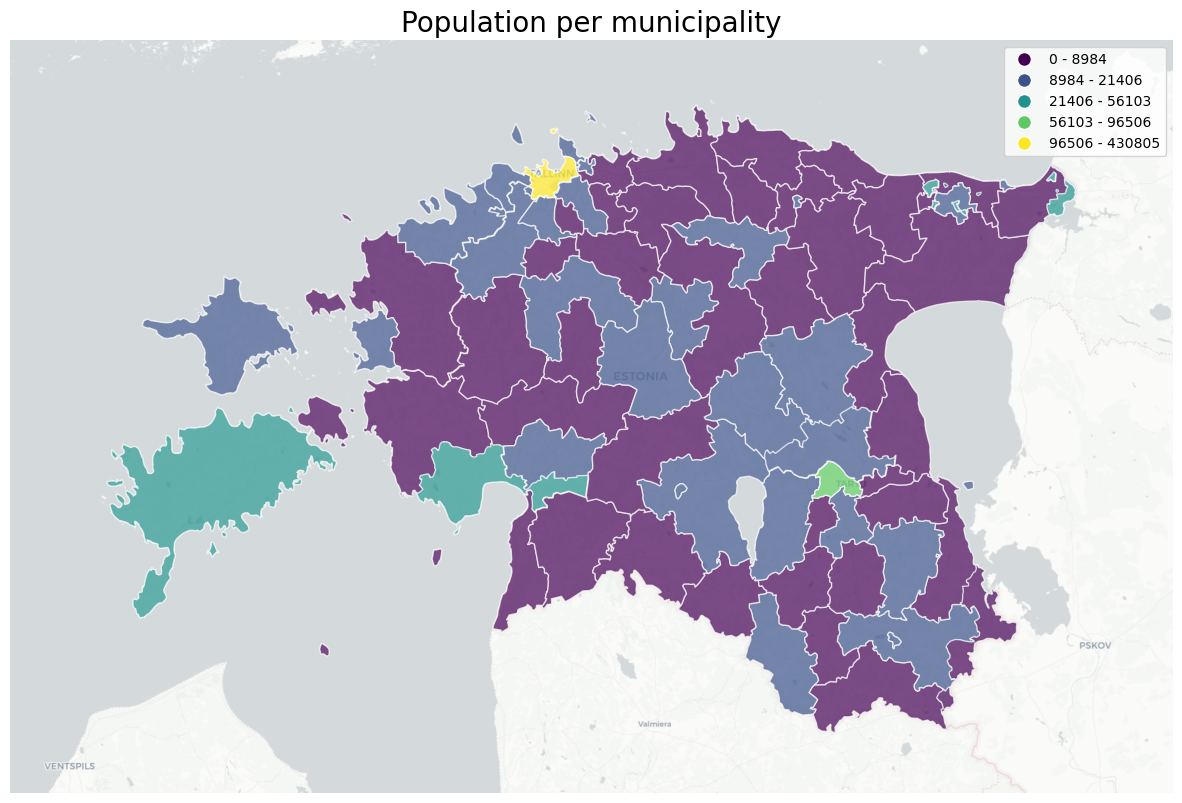GeoPlot cartogram, using a variable as scale for size of polygon

I’d like to demonstrate the cartographic method of a cartogram.

A cartogram distorts (grows or shrinks) polygons on a map according to the magnitude of some input data. A basic cartogram specifies data, a projection, and a scale parameter.

https://residentmario.github.io/geoplot/api_reference.html

Here I also show, that you can have a dark background, is a option to set in matplotlib. STrong colors and contrasts work well on a dark background.

```# set dark background for matplotlib-based plots
plt.style.use('dark_background')

# again, we need to take the ax object with us to the next plot
# we also reuse the scheme mc classifier
# hue tells from which column to take the value
ax = gplt.cartogram(
acc_wgs84, scale='pop_int', projection=gcrs.AlbersEqualArea(),
legend=True, legend_kwargs={'bbox_to_anchor': (1, 0.9)}, legend_var='hue',
hue='pop_int',
scheme=scheme,
cmap='viridis',
limits=(0.5, 1),
figsize=(15,12)
)
# for comparison we also plot the original size boundaries
gplt.polyplot(acc_wgs84, facecolor='white', edgecolor='white', alpha=0.8, ax=ax)

plt.title("Population per municipality as cartogram", fontsize=20)
```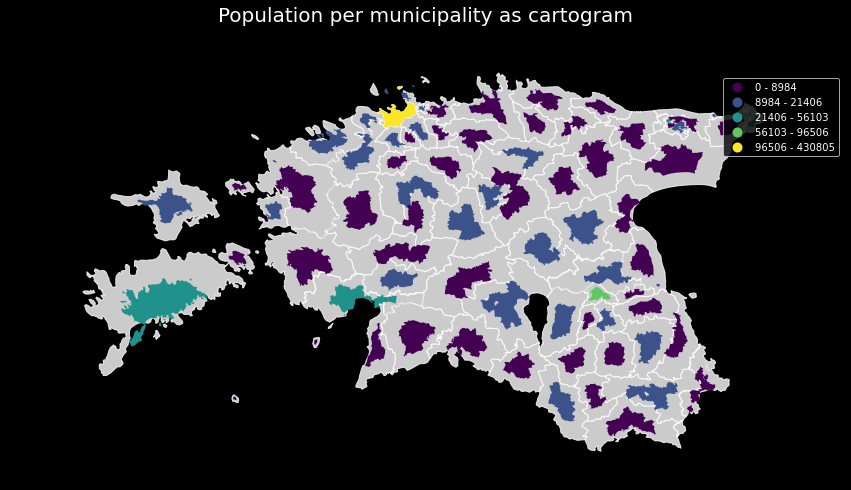GeoPlot making a static KDE plot as contour or filled

Geoplot provides a variety of practical use-case-based plot types. The last one I’d like to show here is the KDEplot.

Kernel density estimation (KDE) is a technique that non-parameterically estimates a distribution function for a sample of point observations. It is not a heatmap as such, as we don’t scale based on parameter values from the point data, but only the number of points close together. KDEs are a popular tool for analyzing data distributions.

A basic kdeplot takes pointwise data as input. For spatial context, let’s also plot an underlying basemap. For completeness we also set title and save the plot.

https://residentmario.github.io/geoplot/api_reference.html

```# reset the background to default / white again
plt.style.use('default')
```

The underlying webmap plot function has a few limitations, for example that it interpretes geomtries as WGS84 rto calculate extent etc. Also, the webmap only plots tiles in WebMercator projection. So we reproject the data to WGS84.

```c4 = catchments.to_crs(4326)
s4 = species_data.to_crs(4326)
```

Now we build up the whole plot example. We can initialize our matplotlib plt handle, but not the ax object. As you see, we have to take the ax object from each function and pass it to the next. With the plt handle we have the context to save the image to a file.

```fig = plt.figure()

proj = gcrs.WebMercator()

ax = gplt.webmap(df=gpd.overlay(c4, s4, how='union'), projection=proj, zoom=10, provider=ctx.providers.Stamen.Toner, figsize=(15,12))

ax = gplt.pointplot(s4, projection=proj, color='green', edgecolor='white',  linewidth=0.5, alpha=0.8, ax=ax)

ax = gplt.kdeplot(s4, projection=proj, cmap='YlOrRd', shade=False, linewidths=5, alpha=0.7, ax=ax)

ax.set_title("Distribution of recorded specimen in south Tartu county", fontsize=18)

plt.savefig("species-kde.png", bbox_inches='tight')
```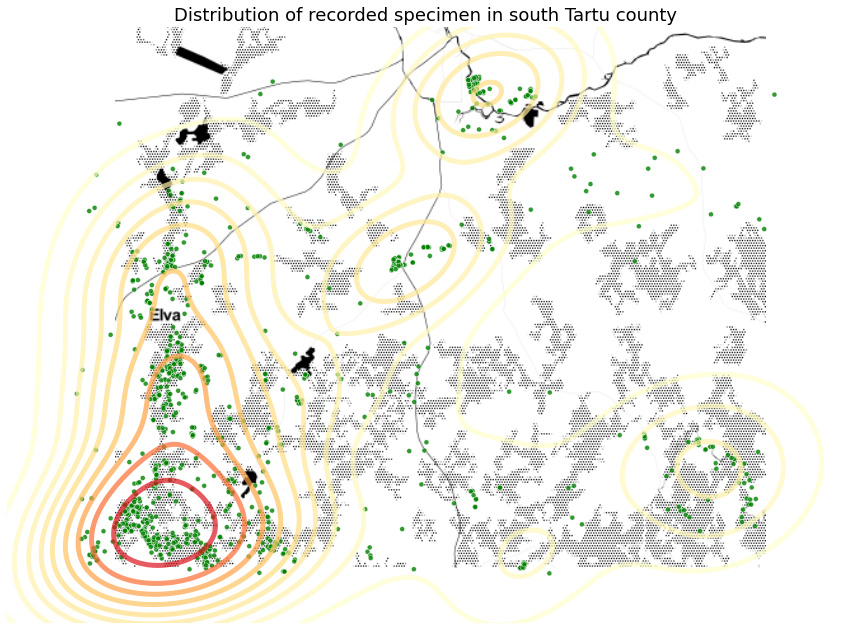The KDEplot function provides a shade parameter, that allows us to switch between contour lines and filled contours.

```proj = gcrs.AlbersEqualArea()

ax = gplt.pointplot(s4, projection=proj, color='green', edgecolor='white',  linewidth=0.5, alpha=0.8, figsize=(15,12))

ax = gplt.polyplot(c4, projection=proj, edgecolor='black',  linewidth=2, alpha=0.7, ax=ax)

ax = gplt.kdeplot(s4, projection=proj, cmap='YlOrRd', shade=True, alpha=0.7, ax=ax)
```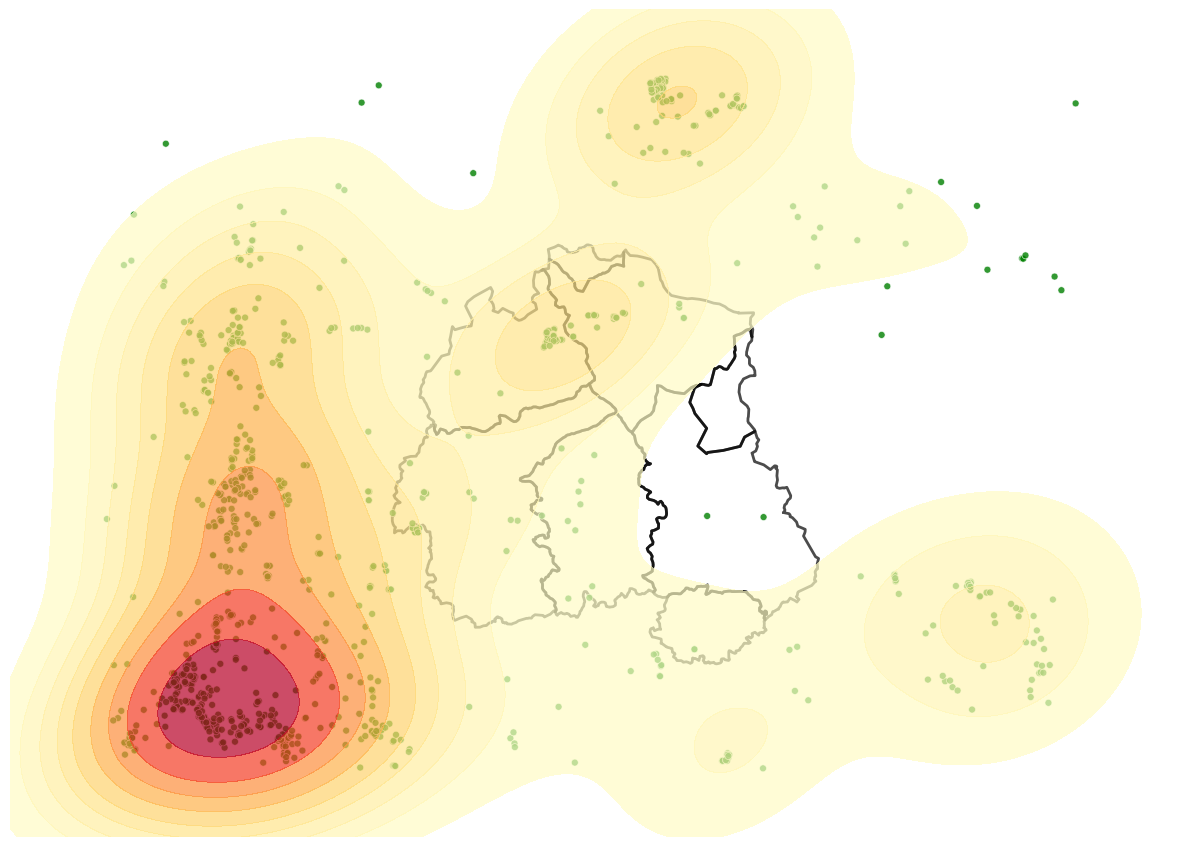Launch in the web/MyBinder: